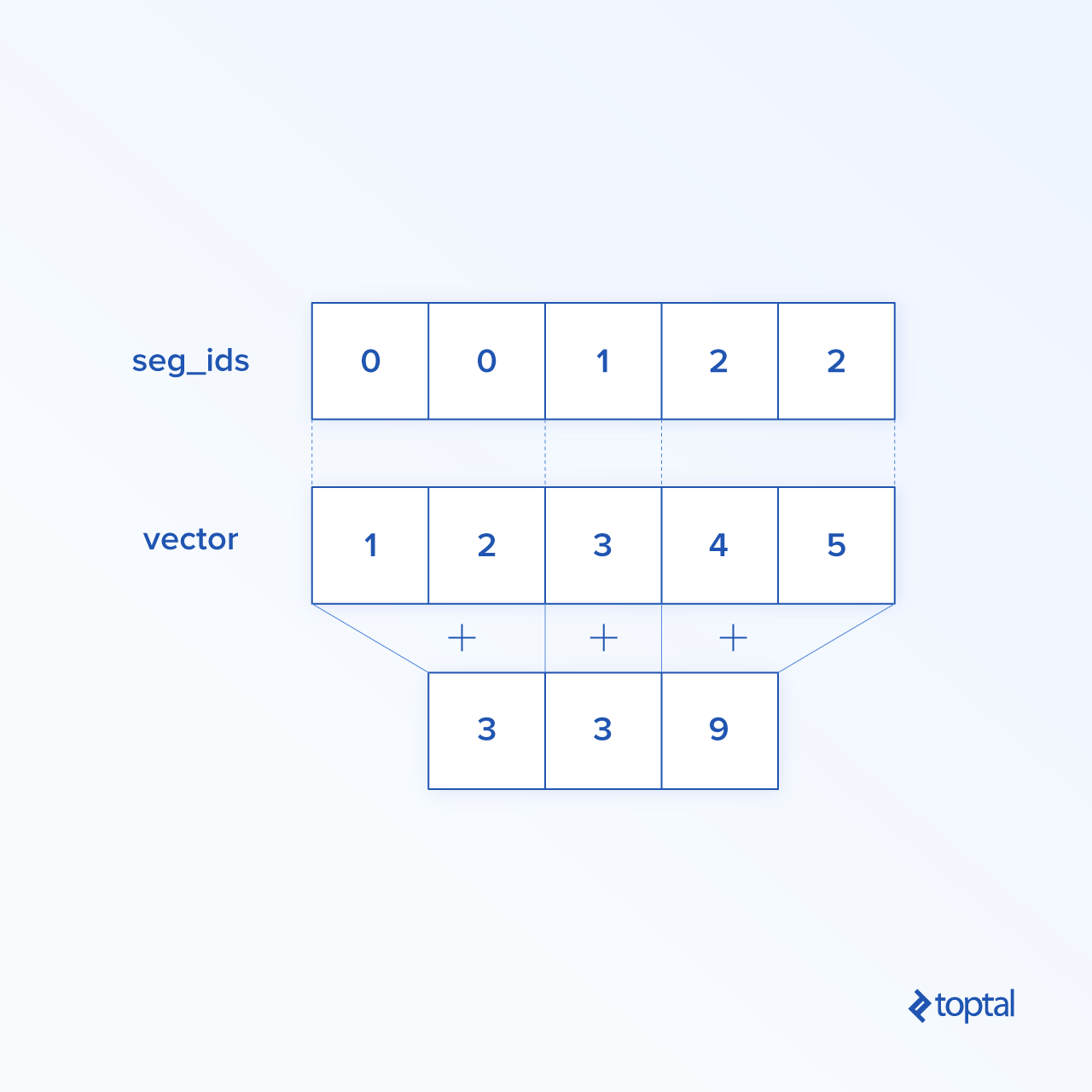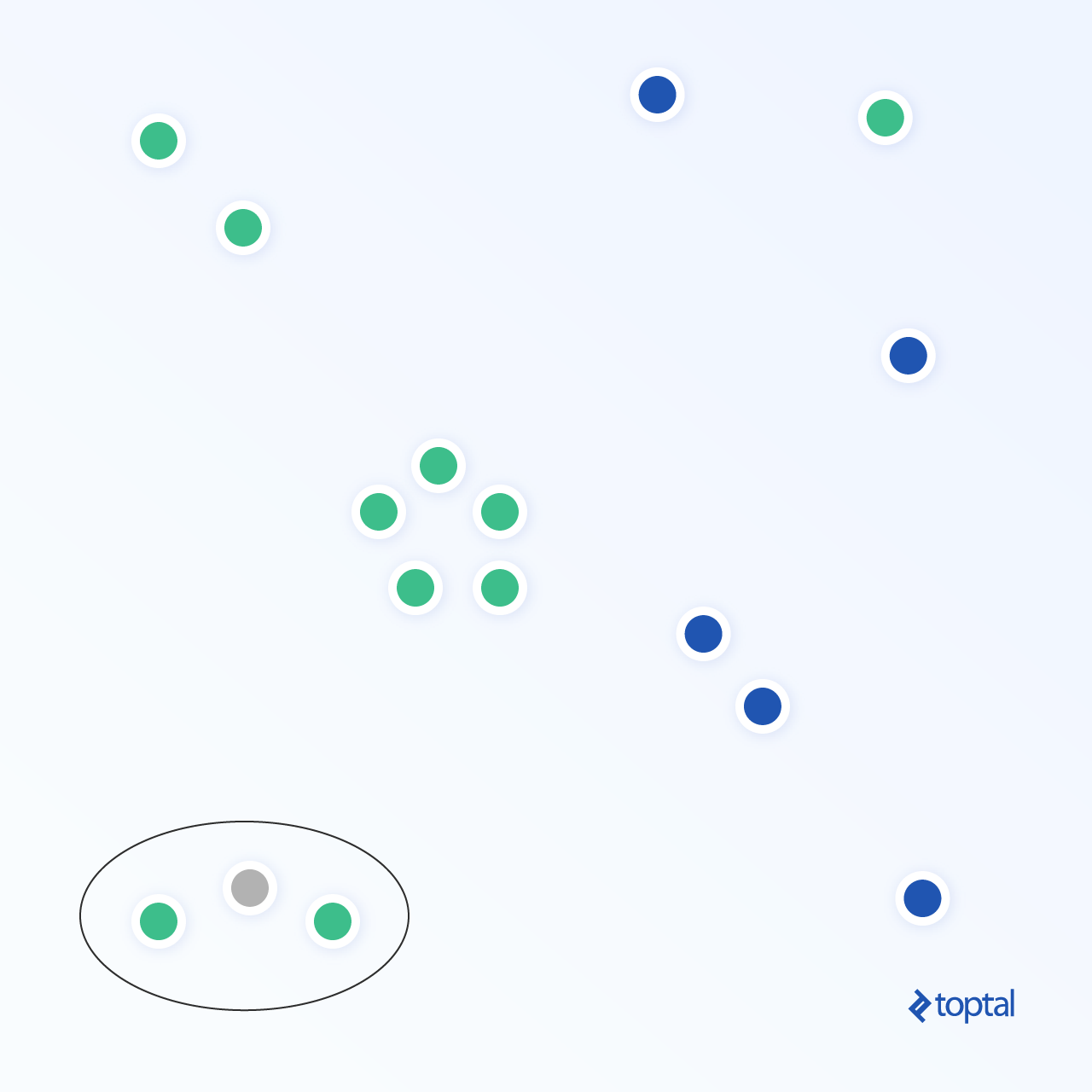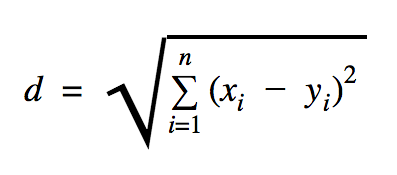Submit a blog to KDnuggets -- Top Blogs Win A Reward

Topics: AI | Data Science | Data Visualization | Deep Learning | Machine Learning | NLP | Python | R | Statistics

KDnuggets Home » News » 2017 » Dec » Tutorials, Overviews » Getting Started with TensorFlow: A Machine Learning Tutorial ( 17:n48 )

# Getting Started with TensorFlow: A Machine Learning Tutorial

A complete and rigorous introduction to Tensorflow. Code along with this tutorial to get started with hands-on examples.

Transforming Data

Reduction

TensorFlow supports different kinds of reduction. Reduction is an operation that removes one or more dimensions from a tensor by performing certain operations across those dimensions. A list of supported reductions for the current version of TensorFlow can be found here. We will present a few of them in the example below.

```import tensorflow as tf
import numpy as np

def convert(v, t=tf.float32):
return tf.convert_to_tensor(v, dtype=t)

x = convert(
np.array(
[
(1, 2, 3),
(4, 5, 6),
(7, 8, 9)
]), tf.int32)

bool_tensor = convert([(True, False, True), (False, False, True), (True, False, False)], tf.bool)

red_sum_0 = tf.reduce_sum(x)
red_sum = tf.reduce_sum(x, axis=1)

red_prod_0 = tf.reduce_prod(x)
red_prod = tf.reduce_prod(x, axis=1)

red_min_0 = tf.reduce_min(x)
red_min = tf.reduce_min(x, axis=1)

red_max_0 = tf.reduce_max(x)
red_max = tf.reduce_max(x, axis=1)

red_mean_0 = tf.reduce_mean(x)
red_mean = tf.reduce_mean(x, axis=1)

red_bool_all_0 = tf.reduce_all(bool_tensor)
red_bool_all = tf.reduce_all(bool_tensor, axis=1)

red_bool_any_0 = tf.reduce_any(bool_tensor)
red_bool_any = tf.reduce_any(bool_tensor, axis=1)

with tf.Session() as session:
print "Reduce sum without passed axis parameter: ", session.run(red_sum_0)
print "Reduce sum with passed axis=1: ", session.run(red_sum)

print "Reduce product without passed axis parameter: ", session.run(red_prod_0)
print "Reduce product with passed axis=1: ", session.run(red_prod)

print "Reduce min without passed axis parameter: ", session.run(red_min_0)
print "Reduce min with passed axis=1: ", session.run(red_min)

print "Reduce max without passed axis parameter: ", session.run(red_max_0)
print "Reduce max with passed axis=1: ", session.run(red_max)

print "Reduce mean without passed axis parameter: ", session.run(red_mean_0)
print "Reduce mean with passed axis=1: ", session.run(red_mean)

print "Reduce bool all without passed axis parameter: ", session.run(red_bool_all_0)
print "Reduce bool all with passed axis=1: ", session.run(red_bool_all)

print "Reduce bool any without passed axis parameter: ", session.run(red_bool_any_0)
print "Reduce bool any with passed axis=1: ", session.run(red_bool_any)
```

Output:

```Reduce sum without passed axis parameter:  45
Reduce sum with passed axis=1:  [ 6 15 24]
Reduce product without passed axis parameter:  362880
Reduce product with passed axis=1:  [  6 120 504]
Reduce min without passed axis parameter:  1
Reduce min with passed axis=1:  [1 4 7]
Reduce max without passed axis parameter:  9
Reduce max with passed axis=1:  [3 6 9]
Reduce mean without passed axis parameter:  5
Reduce mean with passed axis=1:  [2 5 8]
Reduce bool all without passed axis parameter:  False
Reduce bool all with passed axis=1:  [False False False]
Reduce bool any without passed axis parameter:  True
Reduce bool any with passed axis=1:  [ True  True  True]
```

The first parameter of reduction operators is the tensor that we want to reduce. The second parameter is the indexes of dimensions along which we want to perform the reduction. That parameter is optional, and if not passed, reduction will be performed along all dimensions.

We can take a look at the reduce_sum operation. We pass a 2-d tensor, and want to reduce it along dimension 1.

In our case, the resulting sum would be:

```[1 + 2 + 3 = 6, 4 + 5 + 6 = 15, 7 + 8 + 9 = 24]
```

If we passed dimension 0, the result would be:

```[1 + 4 + 7 = 12, 2 + 5 + 8 = 15, 3 + 6 + 9 = 18]
```

If we don’t pass any axis, the result is just the overall sum of:

```1 + 4 + 7 = 12, 2 + 5 + 8 = 15, 3 + 6 + 9 = 45
```

All reduction functions have a similar interface and are listed in the TensorFlow reduction documentation.

Segmentation
Segmentation is a process in which one of the dimensions is the process of mapping dimensions onto provided segment indexes, and the resulting elements are determined by an index row.

Segmentation is actually grouping the elements under repeated indexes, so for example, in our case, we have segmented ids `[0, 0, 1, 2, 2]` applied on tensor `tens1`, meaning that the first and second arrays will be transformed following segmentation operation (in our case summation) and will get a new array, which looks like `(2, 8, 1, 0) = (2+0, 5+3, 3-2, -5+5)`. The third element in tensor `tens1` is untouched because it isn’t grouped in any repeated index, and last two arrays are summed in same way as it was the case for the first group. Beside summation, TensorFlow supports product, mean, max, and min.```import tensorflow as tf
import numpy as np

def convert(v, t=tf.float32):
return tf.convert_to_tensor(v, dtype=t)

seg_ids = tf.constant([0, 0, 1, 2, 2])
tens1 = convert(np.array([(2, 5, 3, -5), (0, 3, -2, 5), (4, 3, 5, 3), (6, 1, 4, 0), (6, 1, 4, 0)]), tf.int32)
tens2 = convert(np.array([1, 2, 3, 4, 5]), tf.int32)

seg_sum = tf.segment_sum(tens1, seg_ids)
seg_sum_1 = tf.segment_sum(tens2, seg_ids)

with tf.Session() as session:
print "Segmentation sum tens1: ", session.run(seg_sum)
print "Segmentation sum tens2: ", session.run(seg_sum_1)
```

```Segmentation sum tens1:
[[ 2  8  1  0]
[ 4  3  5  3]
[12  2  8  0]]

Segmentation sum tens2: [3 3 9]
```

Sequence Utilities
Sequence utilities include methods such as:

• argmin function, which returns the index with min value across the axes of the input tensor,
• argmax function, which returns the index with max value across the axes of the input tensor,
• setdiff, which computes the difference between two lists of numbers or strings,
• where function, which will return elements either from two passed elements x or y, which depends on the passed condition, or
• unique function, which will return unique elements in a 1-D tensor.

We demonstrate a few execution examples below:

```import numpy as np
import tensorflow as tf

def convert(v, t=tf.float32):
return tf.convert_to_tensor(v, dtype=t)

x = convert(np.array([
[2, 2, 1, 3],
[4, 5, 6, -1],
[0, 1, 1, -2],
[6, 2, 3, 0]
]))

y = convert(np.array([1, 2, 5, 3, 7]))
z = convert(np.array([1, 0, 4, 6, 2]))

arg_min = tf.argmin(x, 1)
arg_max = tf.argmax(x, 1)
unique = tf.unique(y)
diff = tf.setdiff1d(y, z)

with tf.Session() as session:
print "Argmin = ", session.run(arg_min)
print "Argmax = ", session.run(arg_max)

print "Unique_values = ", session.run(unique)
print "Unique_idx = ", session.run(unique)

print "Setdiff_values = ", session.run(diff)
print "Setdiff_idx = ", session.run(diff)

print session.run(diff)
```

Output:

```Argmin = [2 3 3 3]
Argmax =  [3 2 1 0]
Unique_values =  [ 1.  2.  5.  3.  7.]
Unique_idx =  [0 1 2 3 4]
Setdiff_values =  [ 5.  3.  7.]
Setdiff_idx =  [2 3 4]
```

Machine Learning with TensorFlow

In this section, we will present a machine learning use case with TensorFlow. The first example will be an algorithm for classifying data with the kNN approach, and the second will use the linear regression algorithm.

kNN

The first algorithm is k-Nearest Neighbors (kNN). It’s a supervised learning algorithm that uses distance metrics, for example Euclidean distance, to classify data against training. It is one of the simplest algorithms, but still really powerful for classifying data. Pros of this algorithm:

• Gives high accuracy when the training model is big enough, and
• Isn’t usually sensitive to outliers, and we don’t need to have any assumptions about data.

Cons of this algorithm:

• Computationally expensive, and
• Requires a lot of memory where new classified data need to be added to all initial training instances.The distance which we will use in this code sample is Euclidean, which defines the distance between two points like this:In this formula, `n` is the number of dimensions of the space, `x` is the vector of the training data, and `y` is a new data point that we want to classify.

```import os
import numpy as np
import tensorflow as tf

ccf_train_data = "train_dataset.csv"
ccf_test_data = "test_dataset.csv"

dataset_dir = os.path.abspath(os.path.join(os.path.dirname(__file__), '../datasets'))

ccf_train_filepath = os.path.join(dataset_dir, ccf_train_data)
ccf_test_filepath = os.path.join(dataset_dir, ccf_test_data)

def load_data(filepath):
from numpy import genfromtxt

csv_data = genfromtxt(filepath, delimiter=",", skip_header=1)
data = []
labels = []

for d in csv_data:
data.append(d[:-1])
labels.append(d[-1])

return np.array(data), np.array(labels)

train_dataset, train_labels = load_data(ccf_train_filepath)
test_dataset, test_labels = load_data(ccf_test_filepath)

train_pl = tf.placeholder("float", [None, 28])
test_pl = tf.placeholder("float", )

knn_prediction = tf.reduce_sum(tf.abs(tf.add(train_pl, tf.negative(test_pl))), axis=1)

pred = tf.argmin(knn_prediction, 0)

with tf.Session() as tf_session:
missed = 0

for i in xrange(len(test_dataset)):
knn_index = tf_session.run(pred, feed_dict={train_pl: train_dataset, test_pl: test_dataset[i]})

print "Predicted class {} -- True class {}".format(train_labels[knn_index], test_labels[i])

if train_labels[knn_index] != test_labels[i]:
missed += 1

tf.summary.FileWriter("../samples/article/logs", tf_session.graph)

print "Missed: {} -- Total: {}".format(missed, len(test_dataset))
```

The dataset which we used in above example is one which can be found on the Kaggle datasets section. We used the one which contains transactions made by credit cards of European cardholders. We are using the data without any cleaning or filtering and as per the description in Kaggle for this dataset, it is highly unbalanced. The dataset contains 31 variables: Time, V1, …, V28, Amount, and Class. In this code sample we use only V1, …, V28 and Class. Class labels transactions which are fraudulent with 1 and those which aren’t with 0.

The code sample contains mostly the things which we described in previous sections with exception where we introduced the function for loading a dataset. The function `load_data(filepath)` will take a CSV file as an argument and will return a tuple with data and labels defined in CSV.

Just below that function, we have defined placeholders for the test and trained data. Trained data are used in the prediction model to resolve the labels for the input data that need to be classified. In our case, kNN use Euclidian distance to get the nearest label.

The error rate can be calculated by simple division with the number when a classifier missed by the total number of examples which in our case for this dataset is 0.2 (i.e., the classifier gives us the wrong data label for 20% of test data).

### Linear Regression

The linear regression algorithm looks for a linear relationship between two variables. If we label the dependent variable as y, and the independent variable as x, then we’re trying to estimate the parameters of the function `y = Wx + b`.

Linear regression is a widely used algorithm in the field of applied sciences. This algorithm allows adding in implementation two important concepts of machine learning: Cost function and the gradient descent method for finding the minimum of the function.

A machine learning algorithm that is implemented using this method must predict values of `y` as a function of `x` where a linear regression algorithm will determinate values `W` and `b`, which are actually unknowns and which are determined across training process. A cost function is chosen, and usually the mean square error is used where the gradient descent is the optimization algorithm used to find a local minimum of the cost function.

The gradient descent method is only a local function minimum, but it can be used in the search for a global minimum by randomly choosing a new start point once it has found a local minimum and repeating this process many times. If the number of minima of the function is limited and there are very high number of attempts, then there is a good chance that at some point the global minimum is spotted. Some more details about this technique we will leave for the article which we mentioned in the introduction section.

```import tensorflow as tf
import numpy as np

test_data_size = 2000
iterations = 10000
learn_rate = 0.005

def generate_test_values():
train_x = []
train_y = []

for _ in xrange(test_data_size):
x1 = np.random.rand()
x2 = np.random.rand()
x3 = np.random.rand()
y_f = 2 * x1 + 3 * x2 + 7 * x3 + 4
train_x.append([x1, x2, x3])
train_y.append(y_f)

return np.array(train_x), np.transpose([train_y])

x = tf.placeholder(tf.float32, [None, 3], name="x")
W = tf.Variable(tf.zeros([3, 1]), name="W")
b = tf.Variable(tf.zeros(), name="b")
y = tf.placeholder(tf.float32, [None, 1])

model = tf.add(tf.matmul(x, W), b)

cost = tf.reduce_mean(tf.square(y - model))
train = tf.train.GradientDescentOptimizer(learn_rate).minimize(cost)

train_dataset, train_values = generate_test_values()

init = tf.global_variables_initializer()

with tf.Session() as session:
session.run(init)

for _ in xrange(iterations):

session.run(train, feed_dict={
x: train_dataset,
y: train_values
})

print "cost = {}".format(session.run(cost, feed_dict={
x: train_dataset,
y: train_values
}))

print "W = {}".format(session.run(W))
print "b = {}".format(session.run(b))
```

Output:

```cost = 3.1083032809e-05
W = [[ 1.99049103]
[ 2.9887135 ]
[ 6.98754263]]
b = [ 4.01742554]
```

In the above example, we have two new variables, which we called `cost` and `train`. With those two variables, we defined an optimizer which we want to use in our training model and the function which we want to minimize.

At the end, the output parameters of `W` and `b` should be identical as those defined in the `generate_test_values` function. In line 17, we actually defined a function which we used to generate the linear data points to train where `w1=2`, `w2=3`, `w3=7` and `b=4`. Linear regression from the above example is multivariate where more than one independent variable are used.

Conclusion
As you can see from this TensorFlow tutorial, TensorFlow is a powerful framework that makes working with mathematical expressions and multi-dimensional arrays a breeze—something fundamentally necessary in machine learning. It also abstracts away the complexities of executing the data graphs and scaling.

Over time, TensorFlow has grown in popularity and is now being used by developers for solving problems using deep learning methods for image recognition, video detection, text processing like sentiment analysis, etc. Like any other library, you may need some time to get used to the concepts that TensorFlow is built on. And, once you do, with the help of documentation and community support, representing problems as data graphs and solving them with TensorFlow can make machine learning at scale a less tedious process.

Original. Reposted with permission.

Bio: Dino has over five years of experience as a software developer. For the past two years, he has worked in Java and related technologies, mostly in implementing big data solutions using NoSQL technologies and in implementing REST services. He is based in Sarajevo and is a member of Toptal.

Related

## Top Stories Past 30 Days

Most PopularGet KDnuggets, a leading newsletter on AI, Data Science, and Machine Learning

By subscribing you accept KDnuggets Privacy Policy